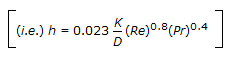Courses

# Test: Heat Transfer - 7

## 25 Questions MCQ Test Heat Transfer | Test: Heat Transfer - 7

Description
This mock test of Test: Heat Transfer - 7 for Chemical Engineering helps you for every Chemical Engineering entrance exam. This contains 25 Multiple Choice Questions for Chemical Engineering Test: Heat Transfer - 7 (mcq) to study with solutions a complete question bank. The solved questions answers in this Test: Heat Transfer - 7 quiz give you a good mix of easy questions and tough questions. Chemical Engineering students definitely take this Test: Heat Transfer - 7 exercise for a better result in the exam. You can find other Test: Heat Transfer - 7 extra questions, long questions & short questions for Chemical Engineering on EduRev as well by searching above.
QUESTION: 1

Solution:
QUESTION: 2

Solution:
QUESTION: 3

### If the baffle spacing in a shell and tube heat exchanger increases, then the Reynolds number of the shell side fluid

Solution:
QUESTION: 4

The heat transfer by radiation from a mild steel surface is to be reduced by reducing the emissivity of the surface. This can be best achieved by

Solution:
QUESTION: 5

__________ paint has the maximum absorption coefficient.

Solution:
QUESTION: 6

What is Nusselt number ?

Solution:
QUESTION: 7

Steam traps are provided in steam carrying pipelines to

Solution:
QUESTION: 8

The Dietus-Boelter equation for convective leat transfercannot be used for

Solution:
QUESTION: 9

A hot liquid is kept in a big room. The logorithm of the numerical value of the temperature difference between the liquid and the room is plotted against time. The plot will be very nearly a/an

Solution:
QUESTION: 10

Radiation heat transfer rates does not depend upon the

Solution:
QUESTION: 11

Which of the following is correct ?

Solution:
QUESTION: 12

Convective heat transfer, in which heat is transferred by movement of warmed matter is described by

Solution:
QUESTION: 13

As the difference between the wall temperature and bulk temperature increases, the boiling heat transfer co-efficient

Solution:
QUESTION: 14

Heat transfer rate per unit area is called

Solution:
QUESTION: 15

Log mean temperature difference (LMTD) cannot be used, if

Solution:
QUESTION: 16

In a multipass shell and tube heat exchanger, tube side return pressure loss is equal to __________ the velocity head.

Solution:
QUESTION: 17

For a given ambient air temperature with increase in the thickness of insulation of a hot cylinderical pipe, the rate of heat loss from the surface would

Solution:
QUESTION: 18

In a parallel flow heat exchanger, if the outlet temperature of hot and cold fluids are the same, then the log mean temperature difference (LMTD) is

Solution:
QUESTION: 19

For what value of Prandtl number, the Col-burn analogy is valid ?

Solution:
QUESTION: 20

In a forward feed multiple effect, the pressure build up will be

Solution:
QUESTION: 21

Film boiling is usually not desired in commercial equipments, because

Solution:
QUESTION: 22

For the same heat transfer area and the terminal conditions, the ratio of the capacities of a single effect evaporator to a triple effect evaporator is

Solution:
QUESTION: 23

The variation of thermal conductivity of a metal with temperature is often correlated using an expression of the form K = K0 + at. where, K is the thermal conductivity and T is the temperature (in. oK). The units of 'a' in SI system will be

Solution:
QUESTION: 24

Film condensation is promoted on a/an __________ surface.

Solution:
QUESTION: 25

Dietus-Boelter equation cannot be used for molten metals mainly due to its very low

Solution: